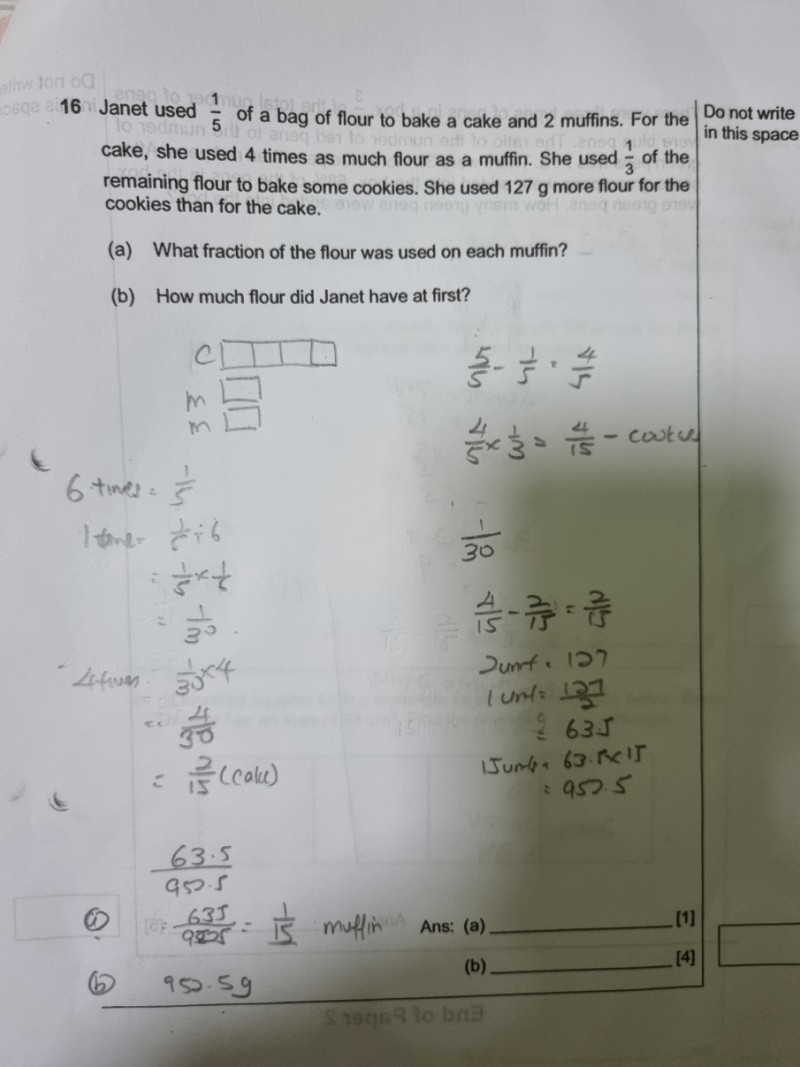# QuestionAre my steps correct.pls help.thanks

Your answer for (a) is for 2 muffins?  If they asked for flour used on muffins, then 1/15 is correct.

Actually you have already worked out 1/30 for each muffin, why did you change answer to 1/15?  You might get method marks but you changed your method to using the mass of flour to get the fraction at the final answer so it might be confusing for the marker.

(b) is correct.

Alternative Solution:

(a)   Cake        [u][u][u][u]   <—-flour used for 1 cake                (total flour used for 1C2M = 1/5)

Muffin    [u][u]               <—-flour used for 2 muffins(cake flour is 4 times as much as a muffin)

Fraction of flour used for each muffin  = (u/6u) x (1/5) = 1/30

(b)  1/3 of remaining flour for cookies = 1/3 x 4/5 = 4/15

Fraction of flour used for cake = (4u/6u) x 1/5 = 2/15

4units – 2 units = 127  => 15 units = (127/2) x 15 = 952.5

Hence flour Janet had at first was 952.5g

0 Replies 1 Like